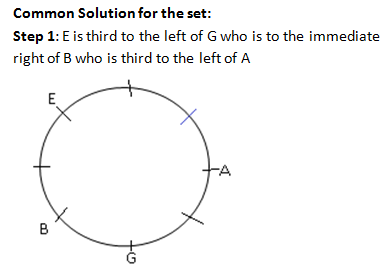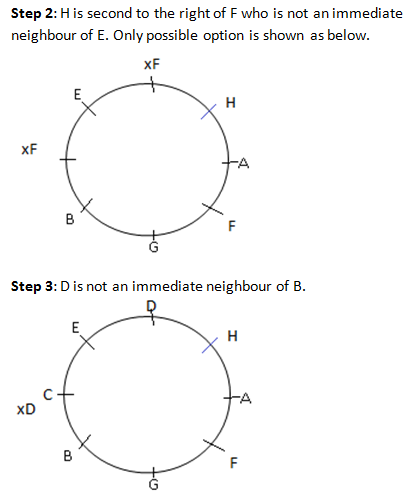### Logical Reasoning definition: Logical Reasoning definition helps you understand the specific areas of Logical reasoning which are tested in the exam

Logical Reasoning definition is: Logical reasoning (which in short means “logic”) is one of the most important skills of effective thinking.
The key to effective problem solving is raising questions like:
• If this is true, what else must be true?
• If this is true, what else is probably true?
• If this isn’t true, what else can’t be true?
The above are connections between 2 or more sentences and are known as inferences. The connections are established between a given sentence (or premise) and another sentence (or conclusion). Inferences are the basic building blocks of logical reasoning, and there are strict rules governing what counts as a valid inference and what doesn’t — it’s a lot like math, but applied to sentences rather than numbers. When you closely read the Logical Reasoning definition given above, you can easily identify the skills that are crucial in this section and that are being tested along with your speed.

Directions for the question set:
Study the following information and answer the questions given below it.
Eight friends A, B, C, D, E, F, G and H are sitting around a circle facing the centre. E is third to the left of G who is to the immediate right of B who is third to the left of A. H is second to the right of F who is not an immediate neighbour of E. D is not an immediate neighbour of B.

Question 1: Who is second to the right of B?
(a) F
(b) A
(c) H
(d) D
(e) None of these

Question 2: Which of the following pairs has the first person to the immediate left of the second person?
(a) GB
(b) AF
(c) CE
(d) HD
(e) None of these

Question 3: Which of the following is the correct position of B with respect to D?
(a) Second to the right
(b) Second to the left
(c) Third to the right
(d) Third to the left
(e) None of these

Question 4: Who sits between A and D?
(a) F
(b) E
(c) G
(d) B
(e) H

Question 5: What is E’s position with respect to C?
(a) To the immediate right
(b) To the immediate left
(c) Second to the right
(d) Cannot be determined
(e) None of these

### Answers and Explanations: Click the down arrow to expandAnswer 1: (a) F is second to the right of B The correct option is (a)

Answer 2: (d) H is to the immediate left of D The correct option is (d)

Answer 3: (c) B is third to the right of D. The correct option is (c)

Answer 4: (e) H is between A and D. The correct option is (e)

Answer 5: (b) E is to the immediate left of C. The correct option is (b)

Extra tips for understanding the Logical Reasoning definition:
• Logical Reasoning definition simply helps you understand in depth the skills that are being tested. Therefore, read the definition to learn the right type of questions you are suppose to ask while working on a solution
• Go through Logical Reasoning definition and list down the specific areas that are tested in logical reasoning. Ensure that checklist all these topics during your preparation.